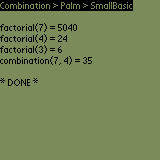Home > Palm > Samples > Combination > BasicPalm sample in Basic

####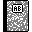Purpose

This is the Basic version of our combination application. It's one of the Palm Samples.

####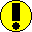Requirements

You need SmallBasic (see tools section).

####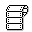Source code

Below is the .bas file.

```' Combination.bas
' Palm
' SmallBasic

func factorial(n)
local total

total = 1

if (n > 1) then
for i = 2 to n
total = total * i
next i
endif

factorial = total
end

func combination(n, p)
combination = factorial(n) / (factorial(n-p)*factorial(p))
end

print cat(3); "Combination > Palm > SmallBasic"; cat(0)
print
print "factorial(7) = "; factorial(7)
print "factorial(4) = "; factorial(4)
print "factorial(3) = "; factorial(3)

print "combination(7, 4) = "; combination(7, 4)
```

####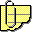Comments

SmallBasic runs on your mobile device.

Copy and Paste the source code above into a new memo with Palm Desktop. Run HotSync. The source code will be stored in a 'Combination.bas memo, that you can load and run with SmallBasic.

The core functions are : factorial(), combination() and the main program.